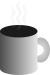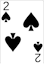#Coffee SpaceListen:

## System Entropy

TL;DR: I tried to do a simulation on a simplified system, and didn’t really prove the point I wanted to make. I think the failure in this regard is worth documenting, though.

There was recently some discussion about the Big Bang theory potentially being rewritten. I’m not sure I am so opinionated on this, but it was interesting. This may or may not have interesting revelations about whether the Universe will undergo a heat death.

One of the assumptions made is that entropy always increases, meaning that over time it becomes “less organized” and more “chaotic”. The assumption here is that the Universe is a closed-system where entropy always increases in an upward trend.

### Entropy

What we mean by entropy here is Shannon entropy, the one defined by information theory, where entropy is defined as:

$H(x) = -\sum_{x \in X} p(x) \log p(x)$

A value of 0 indicates low entropy $H(x)$ and high entropy has a value of 1.

The assumption here is that the Universe started off with an entropy at time $t=0$ that was low, something such as $H(x_{t=0}) \approx 0$, and as time increases and $t \approx \infty$, we see $H(x_{t=\infty}) \approx 1$. (I’ve used approximation as nobody was actually there at the beginning of the Universe or the end, so this is all a little hand-wavy.)

I’m not sure I really agree with this, something tells me this is wrong, but a gut feeling is not nearly good enough to convince anybody, including myself.

### Idea

I don’t have the computation to simulate the Universe, and realistically, I don’t have the computation to even simulate a very simple Universe. What I do have is just about enough computation for a small, ultra-simple Universe.

For this, I chose Langton’s Ant, as it is Turing Complete (this will be important later). It has a very simple rule system and runs pretty fast.

Why this?

1. It’s Turing Complete like the Universe is.
2. It’s based on a simple set of rules that created complex behaviour’s like the Universe is.
3. It starts with low entropy and quickly explodes to have high entropy.
4. Significantly lower computation than anything even remotely resembling a Universe simulation.

As it turned out, I don’t have the computation for this either…

### Experiment

The Universe is setup such that when the agent travels out of bounds, the agent loops back to the other side of the environment. This handles the highway behaviour seen after a set number of cycles. It also means that we don’t need to come up with a specific agent behaviour for the boundary case.

Side note: If did initially try to use a large look-up-table to accelerate the processing, updating the world once the agent exits the kernel, but unfortunately this code was buggy for some reason or another. There was a lot of bit-packing and if just one bit is astray, the entire thing will spew out random system states.

### Running

Of course, I needed to calculate an upper bound for when an solution must be found. It turns out that these numbers end up stupendously large, even for small environments.

So how many runs is that?

Environment length Maximum runs
5 3,355,443,200
6 9,895,604,649,984
7 110,338,190,870,577,152
8 4,722,366,482,869,645,213,696
9 783,383,931,110,279,705,209,602,048
10 507,060,240,091,291,760,598,681,282,150,400

Yeah… I gave up after the first two experiments. Even logging the entropy from these experiments become to arduous.

### Results

For environments of size 5 and 6 in size, the entropy doesn’t significantly reduce after the initial run for the total of the maximum runs. Damn.

As it turns out, despite the problem being Turing Complete, of course this requires certain entry conditions. Otherwise, the most likely thing to occur when executing “random” instructions is that the agent will enter into an infinite loop.

### Future

For the Langton’s Ant problem, I don’t think there is much of a future for exploring the problem. I believe I would need to investigate an alternative version where the number of black and white patches are conserved, as would be most physical properties of the Universe.